×#### Thank you for registering.

One of our academic counsellors will contact you within 1 working day.

Click to Chat

1800-1023-196

+91-120-4616500

CART 0

• 0

MY CART (5)

Use Coupon: CART20 and get 20% off on all online Study Material

ITEM
DETAILS
MRP
DISCOUNT
FINAL PRICE
Total Price: Rs.

There are no items in this cart.
Continue Shopping• Complete JEE Main/Advanced Course and Test Series
• OFFERED PRICE: Rs. 15,900
• View Details

```Chapter 17: Symmetry Exercise 17.4

Question: 1

The total number of lines of symmetry of a scalene triangle is

(a) 1

(b) 2

(c) 3

(d) none of these

Solution:

(d) none of these

This is because the line of symmetry of a scalene triangle is 0.

Question: 2

The total number of lines of symmetry of an isosceles triangle is

(a) 1

(b) 2

(c) 3

(d) none of these

Solution:

(a) 1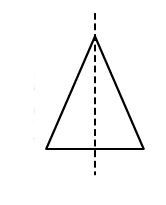Question: 3

An equilateral triangle is symmetrical about each of its

(a) altitudes

(b) median

(c) angle of bisectors

(d) all of the above

Solution:

(d) all the above

In equilateral triangle altitudes, angle bisectors and medians are all the same.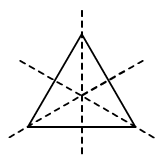Question: 4

The total number of lines of symmetry of a square is

(a) 1

(b) 2

(c) 3

(d) 4

Solution:

(d) 4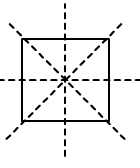Question: 5

(a) each of its diagonals

(b) the line joining the mid-points of its opposite sides

(c) perpendicular bisectors of each of its sides

(d) none of these

Solution:

(a)

Each of its diagonals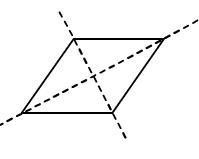Question: 6

The number of lines of symmetry of a rectangle is

(a) 0

(b) 2

(c) 4

(d) 1

Solution:

(b) 2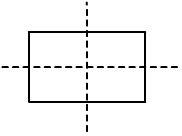Question: 7

The number of lines of symmetry of a kite is

(a) 0

(b) 1

(c) 2

(d) 3

Solution:

(b) 1Question: 8

The number of lines of symmetry of a circle is

(a) 0

(b) 1

(c) 4

(d) unlimited

Solution:

(d) Unlimited

A circle has an infinite number of symmetry all along the diameters. It has an infinite number of diameters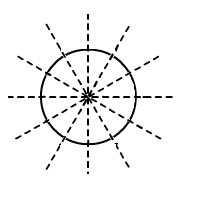Question: 9

The number of lines of symmetry of a regular hexagon is

(a) 1

(b) 2

(c) 6

(d) 8

Solution:

(c) 6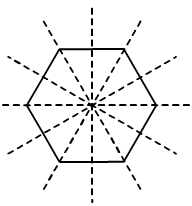Question: 10

The number of lines of symmetry of an n – sided regular polygon is

(a) n

(b) 2n

(c) n/2

(d) none of these

Solution:

(a) n

The number of lines of symmetry of a regular polygon is equal to the sides of the polygon. If it has ‘n’ number of sides, then there are ‘n’ lines of symmetry

Question: 11

The number of lines of symmetry of the letter O of the English alphabet is

(a) 0

(b) 1

(c) 2

(d) 3

Solution:

(c) 2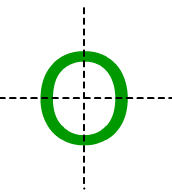Question: 12

The number of lines of symmetry of the letter Z of the English alphabet is

(a) 0

(b) 1

(c) 2

(d) 3

Solution:

(a) 0

Z has no line of symmetry

```### Course Features

• 728 Video Lectures
• Revision Notes
• Previous Year Papers
• Mind Map
• Study Planner
• NCERT Solutions
• Discussion Forum
• Test paper with Video Solution